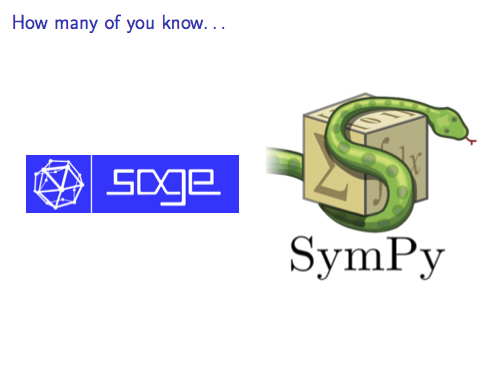# Résolution de problèmes algorithmiques

## Get Started

python3 -m venv venv
. venv/bin/activate  # Load a virtualenv (optional)


But if you don’t need a virtualenv, you can directly do:

pip install sympy
python
>>> from sympy import Symbol, log, exp, pprint
>>> u = sympy.Symbol('u')
>>> v = sympy.Symbol('v')
>>> x = sympy.Symbol('x')
>>> f = x * v * u - log(1 + exp(v * u))
>>> f.diff(u)  # df/du
v*x - v*exp(u*v)/(exp(u*v) + 1)
>>> pprint(f.diff(u))
u⋅v
v⋅ℯ
v⋅x - ────────
u⋅v
ℯ    + 1
>>> pprint(f.diff(u).diff(u))  # d2f/du2
2  u⋅v      2  2⋅u⋅v
v ⋅ℯ        v ⋅ℯ
- ──────── + ───────────
u⋅v                 2
ℯ    + 1   ⎛ u⋅v    ⎞
⎝ℯ    + 1⎠


So if $f = xv^T u - \log(1 + \exp(v^T u))$,

$\frac{\partial^2 f}{ {\partial u}^2 } = \left[\frac{\exp u^T v}{1 + \exp u^T v} + \frac{\exp 2 u^T v}{ {(1 + \exp u^T v)}^2}\right] v v^T.$

(Yes. We cheated.)

## Symbolic computationDifferences between SymPy and SageMath on sympy’s wiki

## SymPy

Try SymPy in your browser (live shell on every page, wow!).

pip install sympy


### SymPy vs. SageMath

SymPy can do better symbolic differentiation than SageMath (ex. derivatives by array). It has symbolic matrices, unlike SageMath. But SageMath has many more libraries, and may be better at factoring expressions, I guess.

### The Matrix Cookbook

What none of these libraries cannot do though, is symbolic matrix differentiation. But it is an ongoing issue on their GitHub.

It made me learn the existence of The Matrix Cookbook, which is a great reference!!

## SageMath

Try it on CoCalc (formerly SageMathCloud).

You can download this SageMath worksheet (logreg.sagews) to load it there.

## But…

But unlike Wolfram Alpha, they cannot output a Pikachu curve :/